# 逻辑学探幽 Part9

## 没有逻辑 只有heip

Posted by R1NG on November 19, 2021 Viewed Times

# 量化布尔公式

## 9.1 量化布尔公式

### 9.1.1 语法和语义

1. 若公式 $F$ 中包含 $\forall p$, 则称在 $F$ 中, $p$ 为 全称量化 (Universally Quantified) 的.

2. 若公式 $F$ 中包含 $\exists p$, 则称在 $F$ 中, $p$ 为 存在量化 (Existentially Quantified) 的.

1. 对 $p$ 的任意可能取值, $F$ 的解释均为真.
2. 对 $p$ 的某一个可能取值, $F$ 的解释为真.

$I[p \leftarrow b](q) \overset{def}{=} \begin{cases} I(q) ~~~ p \neq q \\ b ~~~~~~~~ p = q\end{cases}$

1. $I(\forall p F) = 1$ 当且仅当 $I[p \Leftarrow 0] (F)=1$ 且 $I[p \Leftarrow 1] (F)=1$.
2. $I(\exists p F) = 1$ 当且仅当 $I[p \Leftarrow 0] (F)=1$ 或 $I[p \Leftarrow 1] (F)=1$.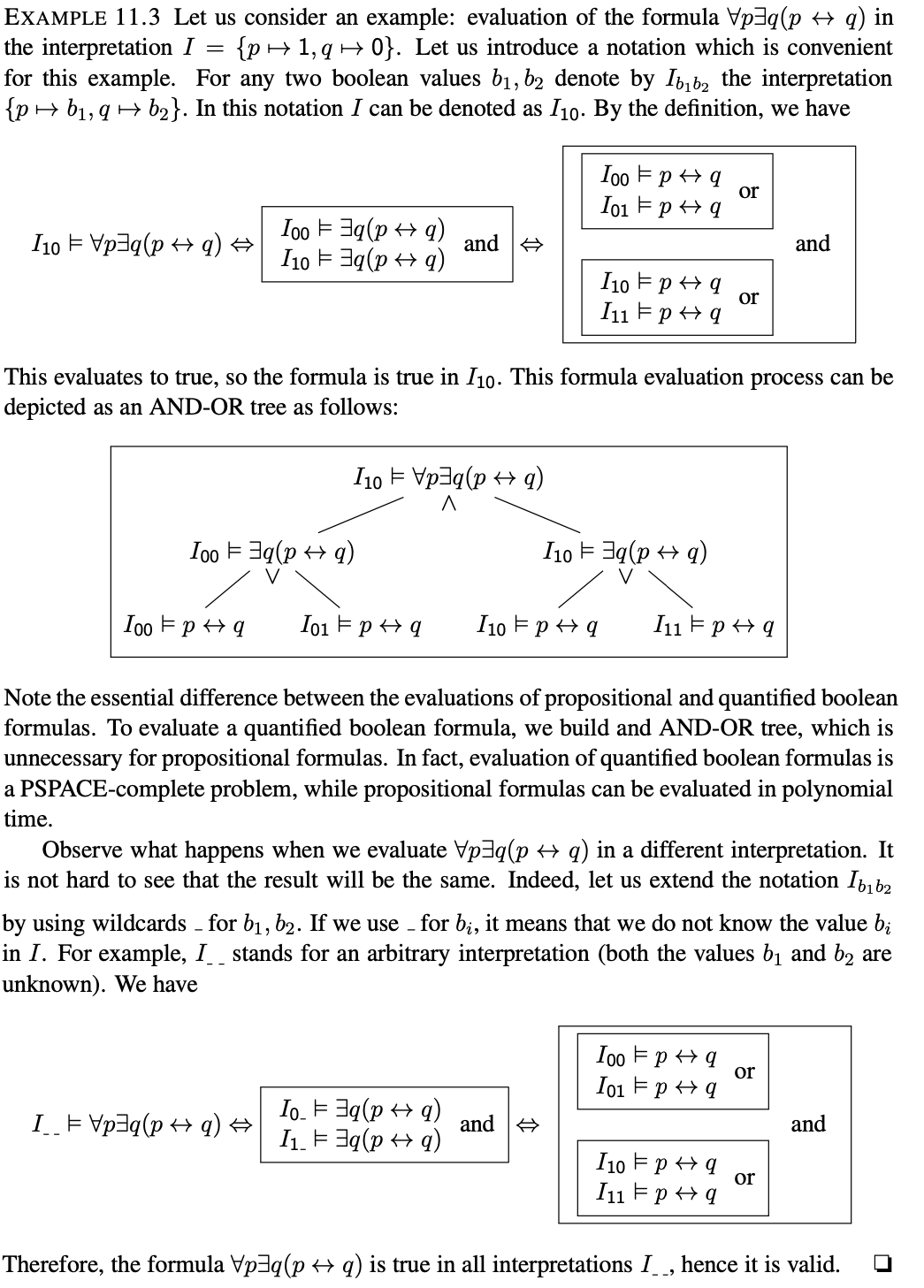### 9.1.2 界限变量和自由变量

$p \rightarrow \forall q \exists p (q \leftrightarrow p) \vee r$

### 9.1.3 真性和满足性

1. $I \vDash F$.
2. $F$ 是可满足的.
3. $F$ 是重言式.

### 9.1.5 代换

1. $F$ 中 不存在既受限又自由 的变量.
2. 对任意变量 $p$, $F$ 最多包涵一个限定它的量词.

## 9.2 范式

### 9.2.1 前束范式

$\exists \forall_{1}p_1 \exists \forall_2 p_2 \cdots \exists \forall_{n} p_n G$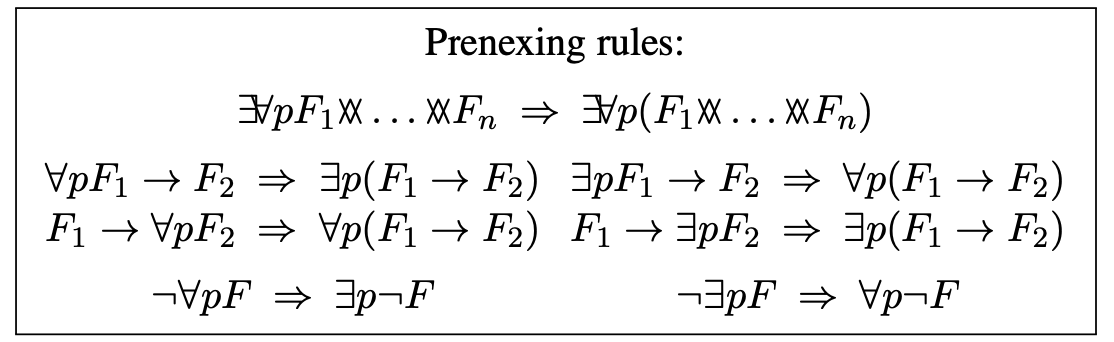### 9.2.2 合取范式

1. $\perp$
2. $\top$
3. $\exists \forall_{1}p_1 \exists \forall_2 p_2 \cdots \exists \forall_{n} p_n F_1$
其中 $n \geqslant 0$ 且 $F_1$ 为某个为合取范式的谓词公式.

## 9.3 可满足性检测: 分割算法和DPLL算法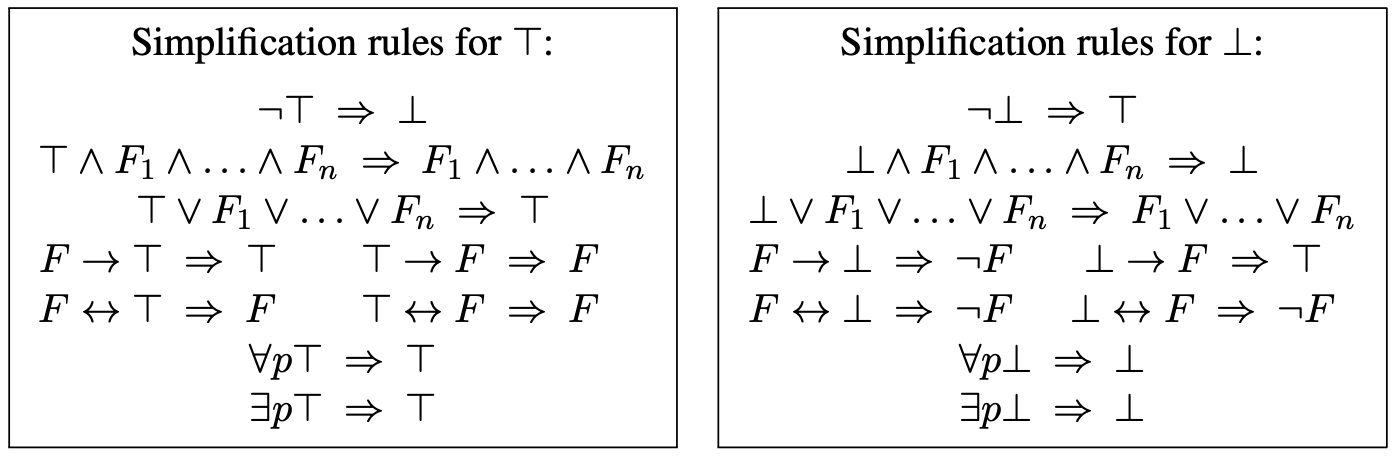1. 称闭的量化布尔公式 $\forall p F$ 为真, 当且仅当 $F_{p}^{\perp}$ 和 $F_{p}^{\top}$ 均为真.
2. 称闭的量化布尔公式 $\exists p F$ 为真, 当且仅当 $F_{p}^{\perp}$ 和 $F_{p}^{\top}$ 至少一个为真.

$\exists \forall_1 p_1 \cdots \exists \forall_n p_n G,$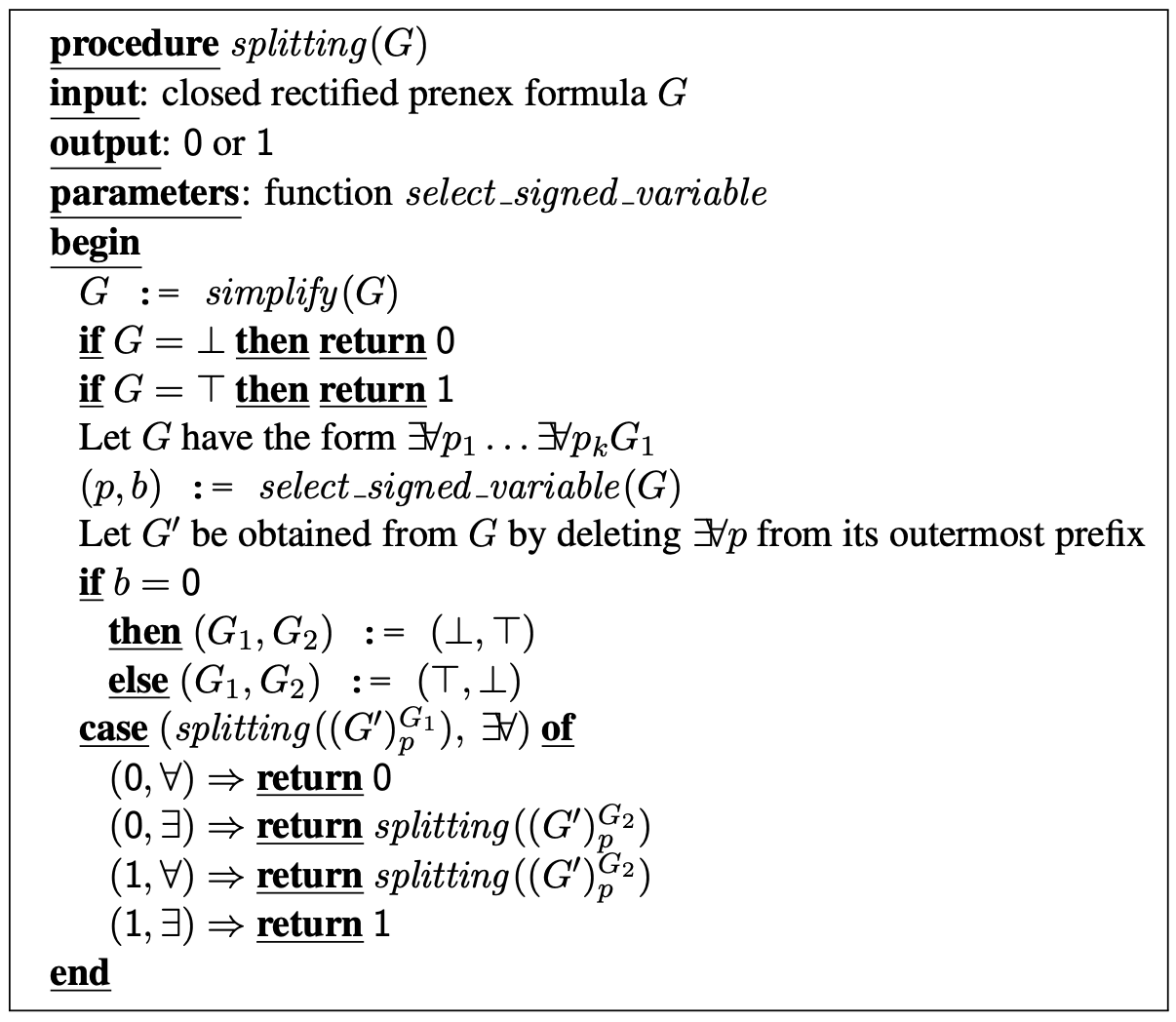1. 若 $F$ 的所有出现均为正, 则 $\exists p F$ 和 $F_{p}^{\top}$ 等价, 且 $\forall p F$ 和 $F_{p}^{\perp}$ 等价.
2. 若 $F$ 的所有出现均为负, 则 $\exists p F$ 和 $F_{p}^{\perp}$ 等价, 且 $\forall p F$ 和 $F_{p}^{\top}$ 等价.

1. 若 $Q$ 包含 $\forall p$, 则将 $S$ 替换为集合 ${\square}$.
2. 否则进行下列操作:
2.1 将 $S$ 中所有形如 $L \vee C’$ 的子句移除.
2.2 将 $S$ 中所有形如 $\widetilde{L} \vee C’$ 的子句取代为 $C’$.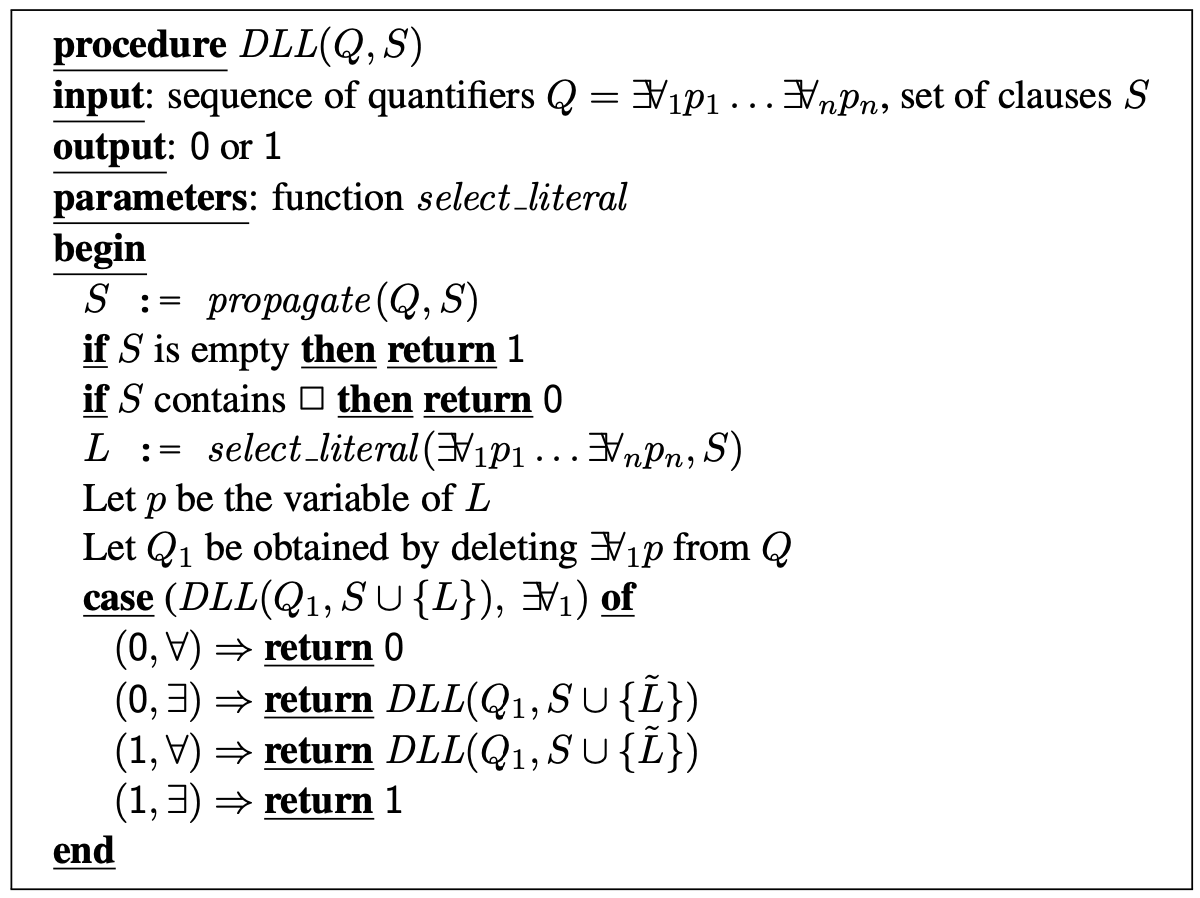$\exists p \forall q \exists r ((p \vee q \vee \neg r) \wedge (p \vee \neg q \vee r) \wedge (\neg p \vee q \vee r) \wedge (\neg p \vee q \neg r))$